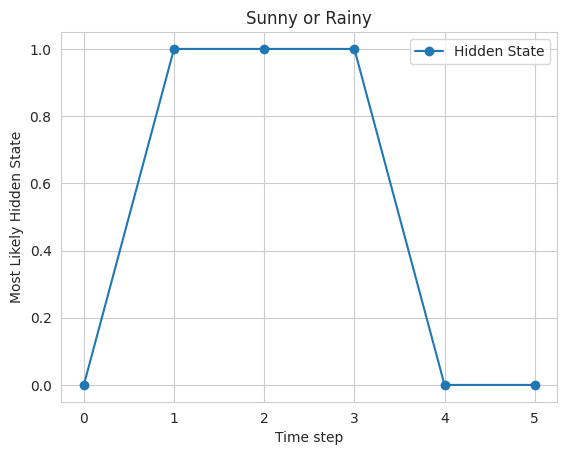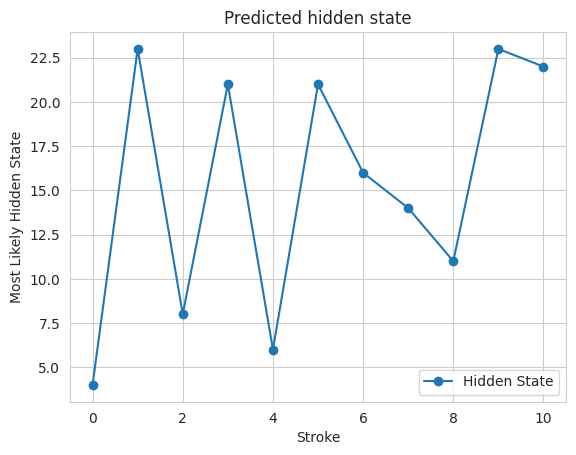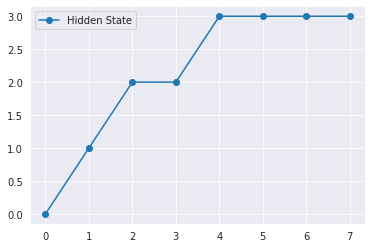# Hidden Markov Model in Machine learning

Hidden Markov Model (HMM) is a statistical model that is used to describe the probabilistic relationship between a sequence of observations and a sequence of hidden states. It is often used in situations where the underlying system or process that generates the observations is unknown or hidden, hence it got the name “Hidden Markov Model.”

It is used to predict future observations or classify sequences, based on the underlying hidden process that generates the data.

An HMM consists of two types of variables: hidden states and observations.

• The hidden states are the underlying variables that generate the observed data, but they are not directly observable.
• The observations are the variables that are measured and observed.

The relationship between the hidden states and the observations is modeled using a probability distribution. The Hidden Markov Model (HMM) is the relationship between the hidden states and the observations using two sets of probabilities: the transition probabilities and the emission probabilities.

• The transition probabilities describe the probability of transitioning from one hidden state to another.
• The emission probabilities describe the probability of observing an output given a hidden state.

### Hidden Markov Model  Algorithm

The Hidden Markov Model (HMM) algorithm can be implemented using the following steps:

#### Step 1: Define the state space and observation space

The state space is the set of all possible hidden states, and the observation space is the set of all possible observations.

#### Step 2:Define the initial state distribution

This is the probability distribution over the initial state.

#### Step 3:Define the state transition probabilities

These are the probabilities of transitioning from one state to another. This forms the transition matrix, which describes the probability of moving from one state to another.

#### Step 4:Define the observation likelihoods:

These are the probabilities of generating each observation from each state. This forms the emission matrix, which describes the probability of generating each observation from each state.

#### Step 5:Train the model

The parameters of the state transition probabilities and the observation likelihoods are estimated using the Baum-Welch algorithm, or the forward-backward algorithm. This is done by iteratively updating the parameters until convergence.

#### Step 6:Decode the most likely sequence of hidden states

Given the observed data, the Viterbi algorithm is used to compute the most likely sequence of hidden states. This can be used to predict future observations, classify sequences, or detect patterns in sequential data.

#### Step 7:Evaluate the model

The performance of the HMM can be evaluated using various metrics, such as accuracy, precision, recall, or F1 score.

To summarise, the HMM algorithm involves defining the state space, observation space, and the parameters of the state transition probabilities and observation likelihoods, training the model using the Baum-Welch algorithm or the forward-backward algorithm, decoding the most likely sequence of hidden states using the Viterbi algorithm, and evaluating the performance of the model.

## Implementation in Python

Key steps in the Python implementation of a simple Hidden Markov Model (HMM) using the hmmlearn library.

### Example 1. Predicting the weather

Problem statement: Given the historical data on weather conditions, the task is to predict the weather for the next day based on the current day’s weather.

#### Step 1: Import the required libraries

The code imports the NumPy,matplotlib, seaborn, and the hmmlearn library.

## Python3

 `# import the necessary libraries ` `import` `numpy as np ` `import` `matplotlib.pyplot as plt ` `import` `seaborn as sns ` `from` `hmmlearn ``import` `hmm`

#### Step 2: Define the model parameters

In this example, The state space is defined as a state which is a list of two possible weather conditions: “Sunny” and “Rainy”. The observation space is defined as observations which is a list of two possible observations: “Dry” and “Wet”. The number of hidden states and the number of observations are defined as constants.

## Python3

 `# Define the state space ` `states ``=` `[``"Sunny"``, ``"Rainy"``] ` `n_states ``=` `len``(states) ` `print``(``'Number of hidden states :'``,n_states) ` `# Define the observation space ` `observations ``=` `[``"Dry"``, ``"Wet"``] ` `n_observations ``=` `len``(observations) ` `print``(``'Number of observations  :'``,n_observations)`

Output:

```Number of hidden states : 2
Number of observations  : 2```

The start probabilities, transition probabilities, and emission probabilities are defined as arrays. The start probabilities represent the probabilities of starting in each of the hidden states, the transition probabilities represent the probabilities of transitioning from one hidden state to another, and the emission probabilities represent the probabilities of observing each of the outputs given a hidden state.

The initial state distribution is defined as state_probability, which is an array of probabilities that represent the probability of the first state being “Sunny” or “Rainy”. The state transition probabilities are defined as transition_probability, which is a 2×2 array representing the probability of transitioning from one state to another. The observation likelihoods are defined as emission_probability, which is a 2×2 array representing the probability of generating each observation from each state.

## Python3

 `# Define the initial state distribution ` `state_probability ``=` `np.array([``0.6``, ``0.4``]) ` `print``(``"State probability: "``, state_probability) ` ` `  `# Define the state transition probabilities ` `transition_probability ``=` `np.array([[``0.7``, ``0.3``], ` `                                   ``[``0.3``, ``0.7``]]) ` `print``(``"\nTransition probability:\n"``, transition_probability) ` `# Define the observation likelihoods ` `emission_probability``=` `np.array([[``0.9``, ``0.1``], ` `                                 ``[``0.2``, ``0.8``]]) ` `print``(``"\nEmission probability:\n"``, emission_probability)`

Output:

```State probability:  [0.6 0.4]

Transition probability:
[[0.7 0.3]
[0.3 0.7]]

Emission probability:
[[0.9 0.1]
[0.2 0.8]]```

#### Step 3: Create an instance of the HMM model and Set the model parameters

The HMM model is defined using the hmm.CategoricalHMM class from the hmmlearn library. An instance of the CategoricalHMM class is created with the number of hidden states set to n_hidden_states and the parameters of the model are set using the startprob_, transmat_, and emissionprob_ attributes to the state probabilities, transition probabilities, and emission probabilities respectively.

## Python3

 `model ``=` `hmm.CategoricalHMM(n_components``=``n_states) ` `model.startprob_ ``=` `state_probability ` `model.transmat_ ``=` `transition_probability ` `model.emissionprob_ ``=` `emission_probability`

#### Step 4: Define an observation sequence

A sequence of observations is defined as a one-dimensional NumPy array.

The observed data is defined as observations_sequence which is a sequence of integers, representing the corresponding observation in the observations list.

## Python3

 `# Define the sequence of observations ` `observations_sequence ``=` `np.array([``0``, ``1``, ``0``, ``1``, ``0``, ``0``]).reshape(``-``1``, ``1``) ` `observations_sequence`

Output:

```array([,
,
,
,
,
])```

#### Step 5: Predict the most likely sequence of hidden states

The most likely sequence of hidden states is computed using the prediction method of the HMM model.

## Python3

 `# Predict the most likely sequence of hidden states ` `hidden_states ``=` `model.predict(observations_sequence) ` `print``(``"Most likely hidden states:"``, hidden_states)`

Output:

`Most likely hidden states: [0 1 1 1 0 0]`

#### Step 6:Decode the observation sequence

The Viterbi algorithm is used to calculate the most likely sequence of hidden states that generated the observations using the decode method of the model. The method returns the log probability of the most likely sequence of hidden states and the sequence of hidden states itself.

## Python3

 `log_probability, hidden_states ``=` `model.decode(observations_sequence, ` `                                              ``lengths ``=` `len``(observations_sequence), ` `                                              ``algorithm ``=``'viterbi'` `) ` ` `  `print``(``'Log Probability :'``,log_probability) ` `print``(``"Most likely hidden states:"``, hidden_states)`

Output:

```Log Probability : -6.360602626270058
Most likely hidden states: [0 1 1 1 0 0]```

This is a simple algo of how to implement a basic HMM and use it to decode an observation sequence. The hmmlearn library provides a more advanced and flexible implementation of HMMs with additional functionality such as parameter estimation and training.

## Python3

 `# Plot the results ` `sns.set_style(``"whitegrid"``) ` `plt.plot(hidden_states, ``'-o'``, label``=``"Hidden State"``) ` `plt.xlabel(``'Time step'``) ` `plt.ylabel(``'Most Likely Hidden State'``) ` `plt.title(``"Sunny or Rainy"``) ` `plt.legend() ` `plt.show()`

Output:Sunny or Rainy

Finally, the results are plotted using the matplotlib library, where the x-axis represents the time steps, and the y-axis represents the hidden state. The plot shows that the model predicts that the weather is mostly sunny, with a few rainy days mixed in.

### Example 2: Handwriting recognition

Problem statement: Given a dataset of handwritten characters, the task is to recognize the character based on its shape.

In this example, the state space is defined as states, which is a list of 26 possible characters. The observation space is defined as observations, which is a list of 8 possible observations, representing the direction of the stroke in the handwriting. The initial state distribution is defined as start_probability, which is an array of probabilities that represent the probability of the first state being any of the 26 characters. The state transition probabilities are defined as transition_probability, which is a 26×26 array representing the probability of transitioning from one character to another. The observation likelihoods are defined as emission_probability, which is a 26×8 array representing the probability of generating each observation from each character.

The HMM model is defined using the hmm.MultinomialHMM class from the hmmlearn library and the parameters of the model are set using the startprob_, transmat_, and emissionprob_ attributes of the model object.

The sequence of observations is defined as observations_sequence and is an array of length 11, representing the 11 strokes in the handwriting. The predict method of the model object is used to predict the most likely hidden states, given the observations. The result is stored in the hidden_states variable, which is an array of length 11, representing the most likely character for each stroke.

## Python3

 `# import the necessary libraries ` `import` `numpy as np ` `import` `matplotlib.pyplot as plt ` `import` `seaborn as sns ` `from` `hmmlearn ``import` `hmm ` ` `  `# Define the state space ` `states ``=` `[``"A"``, ``"B"``, ``"C"``, ``"D"``, ``"E"``, ``"F"``, ``"G"``, ``"H"``, ``"I"``, ``"J"``, ``"K"``, ``"L"``, ``"M"``, ` `          ``"N"``, ``"O"``, ``"P"``, ``"Q"``, ``"R"``, ``"S"``, ``"T"``, ``"U"``, ``"V"``, ``"W"``, ``"X"``, ``"Y"``, ``"Z"``] ` `n_states ``=` `len``(states) ` ` `  ` `  `# Define the observation space ` `observations ``=` `[``"up"``, ``"down"``, ``"left"``, ``"right"``, ``"up-right"``, ``"down-right"``, ` `                ``"down-left"``, ``"up-left"``] ` `n_observations ``=` `len``(observations) ` ` `  ` `  `# Define the initial state distribution ` `start_probability ``=` `np.random.dirichlet(np.ones(``26``),size``=``(``1``))[``0``] ` ` `  `# Define the state transition probabilities ` `transition_probability ``=` `np.random.dirichlet(np.ones(``26``),size``=``(``26``)) ` ` `  ` `  `# Define the observation likelihoods ` `emission_probability ``=` `np.random.dirichlet(np.ones(``26``),size``=``(``26``)) ` ` `  `# Fit the model ` `model ``=` `hmm.CategoricalHMM(n_components``=``n_states) ` `model.startprob_ ``=` `start_probability ` `model.transmat_ ``=` `transition_probability ` `model.emissionprob_ ``=` `emission_probability ` ` `  `# Define the sequence of observations ` `observations_sequence ``=` `np.array([``0``, ``1``, ``2``, ``1``, ``0``, ``1``, ``2``, ``3``, ``2``, ``1``, ``2``]).reshape(``-``1``, ``1``) ` ` `  `# Predict the most likely hidden states ` `hidden_states ``=` `model.predict(observations_sequence) ` `print``(``"Most likely hidden states:"``, hidden_states) ` ` `  `# Plot the results ` `sns.set_style(``"whitegrid"``) ` `plt.plot(hidden_states, ``'-o'``, label``=``"Hidden State"``) ` `plt.xlabel(``'Stroke'``) ` `plt.ylabel(``'Most Likely Hidden State'``) ` `plt.title(``"Predicted hidden state"``) ` `plt.legend() ` `plt.show()`

Output:

`Most likely hidden states: [ 4 23  8 21  6 21 16 14 11 23 22]`Predicted Hidden State

Finally, the results are plotted using the plot function from matplotlib, which shows the predicted hidden state for each stroke in the handwriting.

### Example 3: Speech recognition

Problem statement: Given a dataset of audio recordings, the task is to recognize the words spoken in the recordings.

In this example, the state space is defined as states, which is a list of 4 possible states representing silence or the presence of one of 3 different words. The observation space is defined as observations, which is a list of 2 possible observations, representing the volume of the speech. The initial state distribution is defined as start_probability, which is an array of probabilities of length 4 representing the probability of each state being the initial state.

The state transition probabilities are defined as transition_probability, which is a 4×4 matrix representing the probability of transitioning from one state to another. The observation likelihoods are defined as emission_probability, which is a 4×2 matrix representing the probability of emitting an observation for each state.

The model is defined using the MultinomialHMM class from hmmlearn library and is fit using the startprob_, transmat_, and emissionprob_ attributes. The sequence of observations is defined as observations_sequence and is an array of length 8, representing the volume of the speech in 8 different time steps.

The predict method of the model object is used to predict the most likely hidden states, given the observations. The result is stored in the hidden_states variable, which is an array of length 8, representing the most likely state for each time step.

## Python3

 `import` `numpy as np ` `import` `matplotlib.pyplot as plt ` `import` `seaborn as sns ` `from` `hmmlearn ``import` `hmm ` ` `  `# Define the state space ` `states ``=` `[``"Silence"``, ``"Word1"``, ``"Word2"``, ``"Word3"``] ` `n_states ``=` `len``(states) ` ` `  `# Define the observation space ` `observations ``=` `[``"Loud"``, ``"Soft"``] ` `n_observations ``=` `len``(observations) ` ` `  `# Define the initial state distribution ` `start_probability ``=` `np.array([``0.8``, ``0.1``, ``0.1``, ``0.0``]) ` ` `  `# Define the state transition probabilities ` `transition_probability ``=` `np.array([[``0.7``, ``0.2``, ``0.1``, ``0.0``], ` `                                    ``[``0.0``, ``0.6``, ``0.4``, ``0.0``], ` `                                    ``[``0.0``, ``0.0``, ``0.6``, ``0.4``], ` `                                    ``[``0.0``, ``0.0``, ``0.0``, ``1.0``]]) ` ` `  `# Define the observation likelihoods ` `emission_probability ``=` `np.array([[``0.7``, ``0.3``], ` `                                  ``[``0.4``, ``0.6``], ` `                                  ``[``0.6``, ``0.4``], ` `                                  ``[``0.3``, ``0.7``]]) ` ` `  `# Fit the model ` `model ``=` `hmm.CategoricalHMM(n_components``=``n_states) ` `model.startprob_ ``=` `start_probability ` `model.transmat_ ``=` `transition_probability ` `model.emissionprob_ ``=` `emission_probability ` ` `  `# Define the sequence of observations ` `observations_sequence ``=` `np.array([``0``, ``1``, ``0``, ``0``, ``1``, ``1``, ``0``, ``1``]).reshape(``-``1``, ``1``) ` ` `  `# Predict the most likely hidden states ` `hidden_states ``=` `model.predict(observations_sequence) ` `print``(``"Most likely hidden states:"``, hidden_states) ` ` `  `# Plot the results ` `sns.set_style(``"darkgrid"``) ` `plt.plot(hidden_states, ``'-o'``, label``=``"Hidden State"``) ` `plt.legend() ` `plt.show() `

Output:

`Most likely hidden states: [0 1 2 2 3 3 3 3]`Speech Recognition

## Other Applications of Hidden Markov Model

HMMs are widely used in a variety of applications such as speech recognition, natural language processing, computational biology, and finance. In speech recognition, for example, an HMM can be used to model the underlying sounds or phonemes that generate the speech signal, and the observations could be the features extracted from the speech signal. In computational biology, an HMM can be used to model the evolution of a protein or DNA sequence, and the observations could be the sequence of amino acids or nucleotides.

## Summary

In summary, HMMs are a powerful tool for modeling sequential data, and their implementation through libraries such as hmmlearn makes them accessible and useful for a variety of applications.

Whether you're preparing for your first job interview or aiming to upskill in this ever-evolving tech landscape, GeeksforGeeks Courses are your key to success. We provide top-quality content at affordable prices, all geared towards accelerating your growth in a time-bound manner. Join the millions we've already empowered, and we're here to do the same for you. Don't miss out - check it out now!

Previous
Next